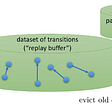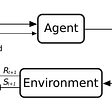# Write an AI to win at Pong from scratch with Reinforcement Learning

• Write a Neural Network from scratch.
• Implement a Policy Gradient with Reinforcement Learning.
• Build an AI for Pong that can beat the computer in less than 250 lines of Python.
• Use OpenAI gym.

## Sources

• Basic Python
• Neural Network design and backpropogation
• Calculus and Linear Algebra

## Setup

1. Open up the code to follow along.
2. Follow the instructions for installing OpenAI Gym. You may need to install cmake first.
3. Run pip install -e .[atari]
4. Go back to the root of this repository and open a new file called my_pong.py in your favorite editor.
5. Let’s get to problem solving. Here’s the problem:

## Problem

• A sequence of images (frames) representing each frame of the Pong game.
• An indication when we’ve won or lost the game.
• An opponent agent that is the traditional Pong computer player.
• An agent we control that we can tell to move up or down at each frame.

## Solution

1. Take in images from the game and preprocess them (remove color, background, downsample etc.).
2. Use the Neural Network to compute a probability of moving up.
3. Sample from that probability distribution and tell the agent to move up or down.
4. If the round is over (you missed the ball or the opponent missed the ball), find whether you won or lost.
5. When the episode has finished(someone got to 21 points), pass the result through the backpropagation algorithm to compute the gradient for our weights.
6. After 10 episodes have finished, sum up the gradient and move the weights in the direction of the gradient.
7. Repeat this process until our weights are tuned to the point where we can beat the computer. That’s basically it! Let’s start looking at how our code achieves this.

# Code

`def main():`

## Initialization

• batch_size: how many rounds we play before updating the weights of our network.
• gamma: The discount factor we use to discount the effect of old actions on the final result. (Don’t worry about this yet)
• num_hidden_layer_neurons: How many neurons are in our hidden layer.
• learning_rate: The rate at which we learn from our results to compute the new weights. A higher rate means we react more to results and a lower rate means we don’t react as strongly to each result.

## Figuring out how to move

1. Crop the image (we just care about the parts with information we care about).
2. Downsample the image.
3. Convert the image to black and white (color is not particularly important to us).
4. Remove the background.
5. Convert from an 80 x 80 matrix of values to 6400 x 1 matrix (flatten the matrix so it’s easier to use).
6. Store just the difference between the current frame and the previous frame if we know the previous frame (we only care about what’s changed).
1. Compute the unprocessed hidden layer values by simply finding the dot product of the weights (weights of layer 1) and the observation_matrix. If you remember, weights is a 200 x 6400 matrix and observations_matrix is a 6400 x 1 matrix. So the dot product will give us a matrix of dimensions 200 x 1. We have 200 neurons so each row represents the output of one neuron.
2. Next, we apply a non linear thresholding function on those hidden layer values - in this case just a simple ReLU. At a high level, this introduces the nonlinearities that makes our network capable of computing nonlinear functions rather than just simple linear ones.
3. We use those hidden layer activation values to calculate the output layer values. This is done by a simple dot product of hidden_layer_values (200 x 1) and weights[‘2’] (1 x 200) which yields a single value (1 x 1).
4. Finally, we apply a sigmoid function on this output value so that it’s between 0 and 1 and is therefore a valid probability (probability of going up).

## Learning

• How does changing the output probability (of going up) affect my result of winning the round?
`∂L/∂f = true_label(0 or 1) — predicted_label(0 or 1)`

--

--

--

## More from Dhruv Parthasarathy

@dhruvp. VP Eng @Athelas. MIT Math and CS Undergrad ’13. MIT CS Masters ’14. Previously: Director of AI Programs @ Udacity.

Love podcasts or audiobooks? Learn on the go with our new app.## Dhruv Parthasarathy

@dhruvp. VP Eng @Athelas. MIT Math and CS Undergrad ’13. MIT CS Masters ’14. Previously: Director of AI Programs @ Udacity.

## Reinforcement Learning: from trial & error to deep Q-learning## Reinforcement Learning: Agent vs Environment## 5 Deep reinforcement learning with Q-functions## Safe Deep Reinforcement Learning